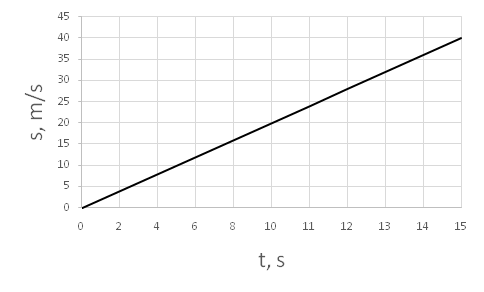• The learning process is entertaining and exciting
• The solved tasks turn into coordinates
• Along with your schoolmates and friends, go on an adventure, searching for hideouts
• E-mail:

# 2939.2. Movement diagram.

#### Given diagram, in which are illustrated, how body movement changes in time. Determine, how long body will move 10 m!s

#### Next task: 2938. Movement diagram.

8
Subject
Physics
Theme
Movement
Subtheme
Movement diagram.

#### Solve tasks to find hidden Geo point coordinates

1. Algebra: Fraction amplification and abbreviation.
2. Algebra: Formula y = k/x
3. Physics: Sounds characteristics.
4. Geometry: Circle in a triangle, centers location.
5. Physics: Lighting and safety.
6. Algebra: Fractions main property
7. Chemistry: Amount of substance.
8. Chemistry: Waters chemical properties and formation.
9. Algebra: Average arithmetical
10. Algebra: Fraction addition and subtraction.